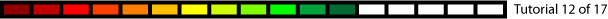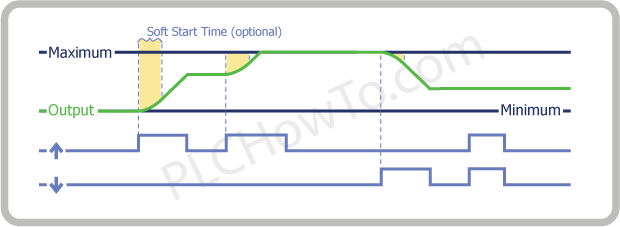# PLC RampA Ramp brings a Output value up or down at a set rate.

Data Used in Simple Ramp:

• Output that will be raised or lowered (Integer or Float)
• Acceleration/Deceleration Rate (Integer or Float).  Typically measured in Units/Sec²
• Acceleration Input
• Deceleration InputIn this example we see that:

• Output Rises when Acceleration Input (Up Arrow) is high
• Output Lowers when Deceleration Input (Down Arrow) is high
• Output remains steady nothing when both Acceleration and Deceleration Input are low or high.
• Though not obvious in this example, Acceleration/Deceleration Rate controls the steepness of the rise & fall in output value.

Now lets look at a more sophisticated Ramp.  We’ll add the following options:

• Maximum & Minimum  (Integer or Float)
• Soft Start Time (Integer or Float) typically measured in ms.  This is the time taken to transition from steady state to Acceleration/Deceleration Rate.  This can reduce the stress on motors.In this example we see:
• Once the Maximum is reached, the Output quits Rising, even when the Acceleration Input is High.  Similar behavior would occur for Minimum if it were included in the example.
• Including Soft Start Time lengthens the amount of time it takes to reach a value.